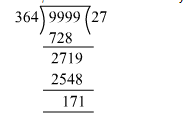# Find the largest four-digits number which when divided by 4, 7 and 13 leaves a remainder of 3 in each case.Question:

Find  the largest four-digits number which when divided by 4, 7 and 13 leaves a remainder of 3 in each case.

Solution:

Largest 4 digit number is 9999
To find the largest 4 digit number divisible by 4, 7 and 13, we find the LCM of 4, 7 and 13 first.

$\operatorname{LCM}(4,7,13)=4 \times 7 \times 13=364$

Now, to we divide 9999 by 364 and subtract the remainder from 9999 to get the number completely divisible by 4, 7 and 13.$9999-171=9828$

Because the number leaves the remainder 3, so we add 3 to 9828.
Therefore, 9828 + 3 = 9831 is the required number.